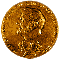Gerard Debreu (1921-)

Premiu Nobel1983

Economist francez, de nationalitate americana, a obtinut Premiul Nobel in Economie in 1983 pentru a fi adus noi metode analitice teoriei economice si pentru riguroasa reformulare a teoriei echilibrului general.Lucrari

"The Coefficient of Resource Utilization", 1951, Econometrica.
"A Social Equilibrium Existence Theorem", 1952,Proceedings of the National Academy of Sciences.
"Definite and Semi-Defnite Quadratic Forms", 1952, Econometrica
"Nonnegative Square Matrices", with I.N. Herstein, 1953, Econometrica.
"Valuation Equilibrium and Pareto Optimum", 1954, Proceedings of the National Academy of Sciences.
"Existence of an Equilibrium for a Competitive Economy", with K.J.Arrow, 1954, Econometrica.

"Representation of a Preference Ordering by a Numerical Function", 1954, in Thrall et al., editors, Decision Processes.
"A Classical Tax-Subsidy Problem", 1954, Econometrica.
"Numerical Representations of Technological Change", 1954, Metroeconomica
"Market Equilibrium", 1956, Proceedings of the NAS.
"Stochastic Choice and Cardinal Utility", 1958, Econometrica
"Cardinal Utility for Even-Chance Mixtures of Pairs of Sure Prospects", 1959, RES
The Theory of Value: An axiomatic analysis of economic equilibrium, 1959
"Topological Methods in Cardinal Utility Theory", 1960, in Arrow, Karlin and Suppes, editors, Mathematical Methods in the Social Sciences.
"On 'An Identity in Arithmetic'", 1960, Proceedings of AMS
"Economics Under Uncertainty", 1960, Economie Appliquee.
"New Concepts and Techniques for Equilibrium Analysis", 1962, IER
"On a Theorem by Scarf", 1963, RES.
"A Limit Theorem on the Core of an Economy", with H.Scarf, 1964, IER.
"Contuinity Properties of Paretian Utility", 1964, IER
"Integration of Correspondences", 1967, Proceedings of Fifth Berkeley Symposium.
"Preference Functions of Measure Spaces of Economic Agents", 1967, Econometrica.
"Neighboring Economic Agents", 1969, La Decision.
"Economies with a Finite Set of Equilibria", 1970, Econometrica.
"Smooth Preferences", 1972, Econometrica.
"The Limit of the Core of an Economy" with H. Scarf", 1972, in McGuire and Radner, editors, Decision and Organization
"Excess Demand Functions", 1974, JMathE
"Four Aspects of the Mathematical Theory of Economic Equilibrium", 1974, Proceedings of Int'l Congress of Mathematicians.
"The Rate of Convergence of the Core of an Economy", 1975, JMathE.
"The Application to Economics of Differential Topology and Global Analysis: Regular differentiable economies", 1976, AER.
"Least Concave Utility Functions", 1976, JMathE.
"Additively Decomposed Quasiconcave Functions", with T.C.Koopmans, 1982, Mathematical Programming.
"Existence of Competitive Equilibrium", 1982, in Arrow and Intriligator, Handbook of Mathematical Econ.
Mathematical Economics: Twenty papers of Gerard Debreu., 1983.
"Economic Theory in a Mathematical Mode: the Nobel lecture", 1984, AER.
"Theoretic Models: Mathematical form and economic content", 1986, Econometrica.
"The Mathematization of Economic Theory", 1991, AER.